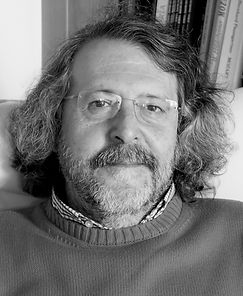top of page

# José M. Mazón

###### RESEARCHERJosé M. Mazón is Full Professor at the Department of Mathematical Analysis at the University of Valencia. He got his Ph. D degree in  Mathematics at the University of Valencia in 1980. Since then his research interests have focused on Mathematical Analysis, with special attention to Partial Differential Equations.  He has been the supervisor of 4 PhD theses and head of 10 research Projects.

In the last 25 years, the main research line of José M. Mazón has been  the study of different problems in Nonlinear PDE’s, mainly concerned with  equations arising in different areas of science such as: image processing, material science and crystal growth, the theory of phase transitions where the corresponding free-energy functional has a linear growth rate with respect to the gradient, nonlinear diffusion problems and the theory of radiation hydrodynamic.

He is the author of more than 100 papers published in prestigious journals. Also, he is the author of two mathematical monographs: “Parabolic Quasilinear Equation Minimizing Linear Growth Functionals”, Birkhauser -Verlag, 2004, in collaboration with F. Andreu and V, Caselles which won the prize Ferran Sunyer i Balaguer 2003, and “Nonlocal Diffusion problem” Math. Surveys and Monographs, 165 (2010), in collaboration with F.Andreu, J. Rossi and J. Toledo.

bottom of page